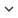ENGArticle
Article 291
Author/s
• I. Rodríguez-Fdez, M. Mucientes , A. Bugarín
DOI
Source
• Information Sciences, 2016 - Q1

# FRULER: Fuzzy Rule Learning through Evolution for Regression

The use of Takagi–Sugeno–Kang (TSK) fuzzy systems in regression problems is widely ex- tended due to the precision of the obtained models. Moreover, the use of simple linear TSK models is usually referred as a good choice in many real problems since it provides a straightforward functional relationship between the output and input variables. In this paper we present FRULER (Fuzzy RUle Learning through Evolution for Regression), a new genetic fuzzy system for automatically learning accurate and simple linguistic TSK fuzzy rule bases for regression problems. FRULER achieves a low complexity of the learned mod- els while keeping a high accuracy, by following three stages: instance selection, multi- granularity fuzzy discretization of the input variables, and evolutionary learning of the rule base combined with Elastic Net regularization to obtain the consequents of the rules. Each of these stages was validated using 28 real-world datasets. FRULER was also experimentally compared with three state of the art genetic fuzzy systems, showing the most accurate and simple models even when compared with approximative approaches.
Keywords: Genetic fuzzy systems, Regression, Instances selection, Multi-granularity fuzzy discretization